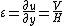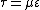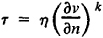# Newtonian Fluid

Also found in: Dictionary, Thesaurus, Medical, Wikipedia.

## Newtonian fluid

A fluid whose stress at each point is linearly proportional to its strain rate at that point. The concept was first deduced by Isaac Newton and is directly analogous to Hooke's law for a solid. All gases are newtonian, as are most common liquids such as water, hydrocarbons, and oils.

A simple example, often used for measuring fluid deformation properties, is the steady one-dimensional flow u(y) between a fixed and a moving wall (see illustration). The no-slip condition at each wall forces the fluid into a uniform shear strain rate ε, given by Eq. (1),

(1)which is induced by a uniform shear stress &tgr;. Here V is the speed of the moving wall, H is the perpendicular distance between the walls, and u is the fluid velocity at distance y from the fixed wall.

If the fluid is newtonian, the experimental plot of &tgr; versus will be a straight line. The constant of proportionality is called the viscosity μ of the fluid, as stated in Eq. (2). (2)The viscosity coefficients of common fluids vary by several orders of magnitude. See Fluid flow, Fluids, Viscosity

McGraw-Hill Concise Encyclopedia of Physics. © 2002 by The McGraw-Hill Companies, Inc.
The following article is from The Great Soviet Encyclopedia (1979). It might be outdated or ideologically biased.

## Newtonian Fluid

a fluid that obeys Newton’s law of viscous friction. For rectilinear laminar flow, this law states that the shear stress τ in the planes of contact of layers of the fluid is directly proportional to the derivative of the rate of flow ν in the direction of the normal n to these planes; that is, τ = η(∂ν/∂n) where η is the coefficient of viscosity. In the general case of a three-dimensional flow, for a Newtonian fluid a linear relation holds between the stress tensor and the tensor of the rates of strain. Most liquids, including water and lubricating oil, and all gases have the properties of a Newtonian fluid. The flow of Newtonian fluids is studied in hydrodynamics and aerodynamics.

Non-Newtonian fluids are fluids for which the relations indicated above are not linear, for example, for the rectilinear flowwhere k ≠ 1. Examples are a number of suspensions and solutions of polymers. Rheology is the study of such flows.

S. M. TARG

## Newtonian fluid

[′nü′tō·nē·ən ′flü·əd]
(fluid mechanics)
A simple fluid in which the state of stress at any point is proportional to the time rate of strain at that point; the proportionality factor is the viscosity coefficient.
McGraw-Hill Dictionary of Scientific & Technical Terms, 6E, Copyright © 2003 by The McGraw-Hill Companies, Inc.
References in periodicals archive ?
Keywords: Newtonian fluid, magnetohydrodynamics, eccentric rotating porous disks, non-torsional oscillation, periodic flow.
Caption: Figure 5: Deformation of the flexible capsule in a shear flow for Reynolds number of Re = 0.05, dimensionless shear rate of G = 0.04, and power-law index of n = 0.2 to 1.8: (a) capsule shapes for difference power-law indices (the dashed line is for the Newtonian fluid case where n = 1.0) and (b) Taylor shape parameter as a function of power-law index.
- It is to mention that when vortex viscosity k and the micro rotation vector are zero, problem of Micropolar fluids corresponding to the Newtonian fluids flow.
(2.) Peralta, JM, Meza, BE, Zorrilla, SE, "Mathematical Modeling of a Dip-Coating Process Using a Generalized Newtonian Fluid. 1.
Viscosity should be written on the left side of the equation in the case of perlite--water mixtures, because they are Newtonian fluids at even high concentrations too.
In this last column, the 0% value had a very high value of [f.sub.T] in order to emulate a Newtonian fluid over the range of frequencies.
The velocity profile in the annular cross-sectional area is flattening around the center and the velocity gradient near the wall is high compared with the Newtonian fluid flow profile.
n is the power-law index, if n < 1 the fluid is said to be pseudo-plastic (shear thinning) fluids, if n > 1 it is called dilatant (thickening) fluids and when n = 1, it is the Newtonian fluid. [sigma] is the electric conductivity, p is the pressure, B is the applied magnetic field strength, T is the temperature of the fluid, [q.sub.r] is the radiative heat flux and [c.sub.p] is the specific heat at constant pressure.
These trends are evidence that once the fiber network strength is overcome by shear stress and turbulence, the mixture behaves as a conventional Newtonian fluid in turbulent flow.
QDPD in its present form is being used to study the steady-shear viscosity of a suspension of solid inclusions (such as ellipsoids) in a Newtonian fluid. The model consists of N particles moving in a continuum domain of volume V.
Theoretically, Non-Newtonian power-law fluid is type of generalized Newtonian fluid for which the apparent viscosity characteristic depends with shear rate.

Site: Follow: Share:
Open / Close